# Lecture 2 - The Local Distance Ladder (1/14/99)Introduction --- | --- Intensity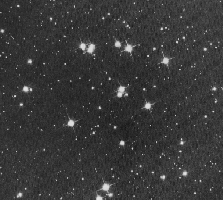Chapter 8-1, 11 (ZG4)

Notes:

pages 3 - 8

Open star cluster M44, the Praesepe (Beehive), in the constellation Cancer. (Courtesy SEDS)
Key Question: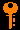## Investigations:

• What is an angular diameter distance and how is it related to a parallax?
• What is a luminosity distance?
• Do angular diameter and luminosity distances to the same object necessarily have to be the same?
• What sort of calibrators are needed to build a useable distance ladder?
• What are the weak rungs in any distance ladder?
2. Flux and Luminosity
• What is the MKS unit of power?
• What does the luminosity of the Sun signify?
• What is a Dyson sphere?
• What is the flux of radiation through a surface?
• How does the solar flux depend on distance?
• What is the solar constant?
3. Magnitude Systems
• Why is it useful to measure brightness or intensity on a logarithmic scale?
• Who first developed the magnitude scale for stars? When was it quantified?
• How are the apparent magnitudes of two stars related to the ratio of their fluxes?
• Does a larger magnitude mean a brighter or fainter star?
• What do we mean by visual, photographic, or U, B, V, etc magnitudes?
• How is the zero of the magnitude system defined?
• How are absolute magnitude and luminosity related?
• How is the absolute magnitude defined in terms of distance and apparent magnitude?
• What is the distance modulus of a star?
• How does the magnitude depend on filter response and intrinsic spectrum?
• What is the UBVRI system?
• What is a color index?
• How is color related to blackbody temperature?
• What sort of star has zero magnitude for all bands?
• What do we mean by bolometric magnitudes?
• What is a bolometric correction?
• What are the apparent visual magnitudes, absolute visual magnitude, bolometric magnitude, and bolometric correction for the Sun?

## Solar Luminosity and Flux:

Every square meter of the Earth's surface receives a certain amount of sunlight, depending of course upon the time of day and the season. Consider a meter-square solar panel, which will convert the sunlight into electrical energy. Alternatively, we could also consider a meter-square panel of black absorbing material that would turn the sunlight into heat. In any event, when oriented perpendicular to the direction toward the Sun, such a panel will intercept the maximum amount of radiant solar energy. This can be measured as power in Watts --- Joules per second. If we were free of the Earth's atmosphere and had an unobstructed view of the Sun at a distance of 1 AU, we would find that we would intercept a particular flux of energy (in Watts per square meter). This is called the solar constant, and has the value of 1370 W/m^2.

Inclining the panel will reduce the intercepted power by the projected area, or

P = F A cos(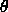)

where F is the solar constant, A the panel area, andthe angle to the perpendicular.

Notice that we could in principle collect all of the Sun's emitted energy by constructing a sphere of radius R= 1 AU enclosing the Sun. Such a sci-fi construction is known as a Dyson sphere and every square meter of its surface would receive a flux equal to the solar constant F. The area of the sphere is 4 Pi R^2, so the Sun must be emitting a power, or luminosity L, of

L = 4 Pi R2 F.

For F=1370 watts/m^2 and R=1 AU=1.5 × 10^11 m, we find that the solar luminosity

Lsun = 4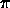(1.5 × 1011 m)2 × (1370 W/m2) = 4 × 1026 W.

Notice that this relation between flux and luminosity will hold for spheres of any radius

F = L / (4R2)

and so the flux falls off as the inverse square of the distance from the source, be it the Sun or a light bulb! For example, the solar flux at the orbit of Mars (R=1.524 AU) will be 0.43 times the solar constant at 1 AU.

## Parallax and Magnitudes:

Finding distances using parallax: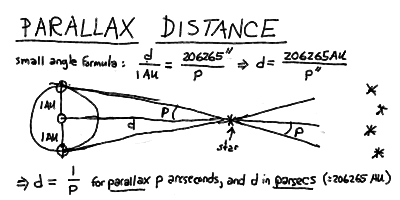Flux (brightness) and luminosity: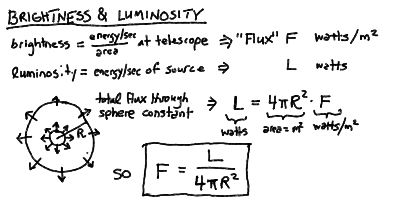Absolute magnitudes and distance modulus: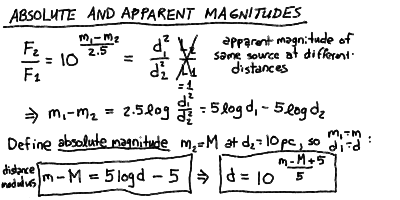The absolute visual magnitude of the Sun: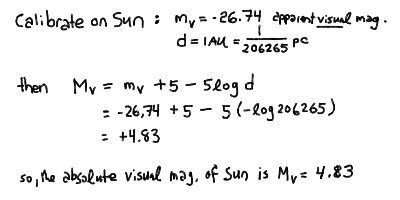Luminosity and absolute visual magnitude: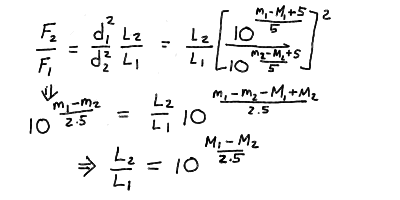Calibrating the relation using the Sun: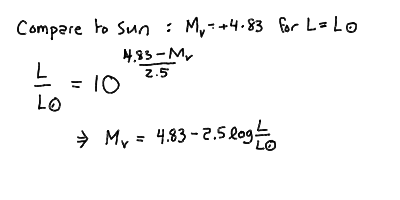## Brightness and Apparent Magnitude:

The Greek astronomer Hipparchus first systematized the visible stars by placing them in six classes ("first class", "second class", etc). This grew into the magnitude system for apparent brightness. Over the 6 magnitude classes that roughly cover the visible stars, the intensity varies by a factor of around 250. This gives rise to the peculiar system where 2.5 magnitudes equals a factor of 10 in brightness, and that larger magnitudes mean fainter stars. This is a logarithmic scale where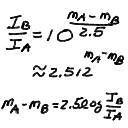gives the relation between the intensities I_A and I_B for two objects A and B with apparent magnitudes m_A and m_B.

Here are the apparent visual magnitudes for some representative astronomical objects:

Object Apparent Magnitude
Sun -26.5
Full Moon -12.5
Venus (at brightest) -4.4
Mars (at brightest) -2.7
Jupiter (at brightest) -2.6
Sirius (brightest star) -1.4
Canopus (second brightest star) -0.7
Vega (zero of scale) 0.0
Spica 1.0
Naked-eye limit (urban areas) 3 - 4
Uranus 5.5
Naked-eye limit (rural areas) 6 - 6.5
Bright Asteroid 6
Neptune 7.8
Limit for typical binoculars 9 - 10
Limit for 15cm (6in) telescope 13
Pluto 15
Hubble Deep Survey 30Prev Lecture ---Next Lecture ---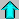Astr12 Index ---Astr12 Home